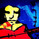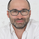‎3005‎ مشاهدة
If you are searching a high win rate strategy with good profit factor ratio strategy. this one may be your choice.
Designed to be used with BTC or LTC. with 5 minute time frame or less. This BB strategy achieve win rate over 50% easily, with some tuning you can get even 60%+.
```//@version=2
strategy(title="yuthavithi BB Scalper 2 strategy", overlay=true)

len = input(20, minval=1, title="Length")
multiplier = input(4, minval=1, title="multiplier")
trendTimeFrame = input(60, minval=1, title="Trend Time Frame")
useTrendFilter = input(true, type=bool, title = "Use Trend Filter")

src = input(close, title="Source")
out = sma(src, len)
//plot(out, title="SMA", color=blue)

stdOut = stdev(close, len)
bbUpper = out + stdOut * multiplier
bbLower = out - stdOut * multiplier
bbUpper2 = out + stdOut * (multiplier / 2)
bbLower2 = out - stdOut * (multiplier / 2)
bbUpperX2 = out + stdOut * multiplier * 2
bbLowerX2 = out - stdOut * multiplier * 2
bbWidth = (bbUpper - bbLower) / out

closeLongTerm = security(tickerid, tostring(trendTimeFrame), close)
smaLongTerm = security(tickerid, tostring(trendTimeFrame), sma(close,20))

//plot(smaLongTerm, color=red)

trendUp = useTrendFilter ? (closeLongTerm > smaLongTerm) : true
trendDown = useTrendFilter? (closeLongTerm < smaLongTerm) : true

bearish = ((cross(close,bbUpper2) == 1) or (cross(close,out) == 1)) and (close > close) and trendDown
bullish = ((cross(close,bbLower2) == 1) or (cross(close,out) == 1)) and (close < close) and trendUp

closeBuy = (high > bbUpper) and (close < bbUpper) and (close < open) and trendUp
closeSell = (((low < bbLower) and (close > bbLower)) or ((low < bbLower) and (close > bbLower))) and (close > open) and trendDown

cutLossBuy = iff(bbWidth > 0.005, (low < bbLower) and (low > bbLower) and trendUp, (low < bbLowerX2) and (low > bbLowerX2) and trendUp)
cutLossSell = iff(bbWidth > 0.005, (high > bbUpper) and (high < bbUpper) and trendDown, (high > bbUpperX2) and (high < bbUpperX2) and trendDown)

if (bullish)

if (bearish)
strategy.entry("Sell", strategy.short, comment="Sell")

strategy.close("Sell", closeSell or cutLossSell)
```

## التعليقاتThis strategy redraws on 3 min time scale. I don't know how but I lost some money trying this out for a couple days. I had a buy signal; three bars later it reversed to a sell signal so i went short then those two both disappeared and a buy signal came out 2 bars after sell. The buy and sell trades had disappeared from list of trades.

Use this at own risk. Author should update that this is a redrawing strategy.
ردdilille010
Trading view must stop providing future data in strategies scripts.
رد